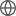Old Web
English
# A Multiwavelength Study of ELAN Environments (AMUSE$^2$). Mass budget, satellites spin alignment and gas infall in a massive $z\sim3$ quasar host halo
The systematic targeting of extended Ly$\alpha$ emission around high-redshift quasars resulted in the discovery of rare and bright Enormous Ly$\alpha$ Nebulae (ELANe) associated with multiple active galactic nuclei (AGN). We here initiate "a multiwavelength study of ELAN environments" (AMUSE$^2$) focusing on the ELAN around the $z\sim3$ quasar SDSS J1040+1020, a.k.a. the Fabulous ELAN. We report on VLT/HAWK-I, APEX/LABOCA, JCMT/SCUBA-2, SMA/850$\mu$m, ALMA/CO(5-4) and 2mm observations and compare them to previously published VLT/MUSE data. The continuum and line detections enable a first estimate of the star-formation rates, dust, stellar and molecular gas masses in four objects associated with the ELAN (three AGNs and one Ly$\alpha$ emitter), confirming that the quasar host is the most star-forming (${\rm SFR}\sim500$ M$_\odot$ yr$^{-1}$) and massive galaxy ($M_{\rm star}\sim10^{11}$ M$_{\odot}$) in the system, and thus can be assumed as central. All four embedded objects have similar molecular gas reservoirs ($M_{\rm H_2}\sim10^{10}$ M$_{\odot}$), resulting in short depletion time scales. This fact together with the estimated total dark-matter halo mass, $M_{\rm DM}=(0.8-2)\times10^{13}$ M$_{\odot}$, implies that this ELAN will evolve into a giant elliptical galaxy. Consistently, the constraint on the baryonic mass budget for the whole system indicates that the majority of baryons should reside in a massive warm-hot reservoir (up to $10^{12}$ M$_{\odot}$), needed to complete the baryons count. Additionally, we discuss signatures of gas infall on the compact objects as traced by Ly$\alpha$ radiative transfer effects and the evidence for the alignment between the satellites' spins and their directions to the central.